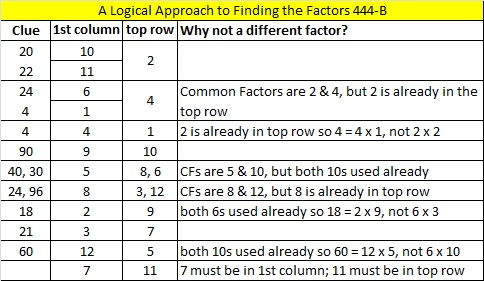# 288 and Level 5

• 288 is a composite number.
• Prime factorization: 288 = 2 x 2 x 2 x 2 x 2 x 3 x 3, which can be written 288 = (2^5) x (3^2)
• The exponents in the prime factorization are 5 and 2. Adding one to each and multiplying we get (5 + 1)(2 + 1) = 6 x 3  = 18. Therefore 288 has exactly 18 factors.
• Factors of 288: 1, 2, 3, 4, 6, 8, 9, 12, 16, 18, 24, 32, 36, 48, 72, 96, 144, 288
• Factor pairs: 288 = 1 x 288, 2 x 144, 3 x 96, 4 x 72, 6 x 48, 8 x 36, 9 x 32, 12 x 24, or 16 x 18
• Taking the factor pair with the largest square number factor, we get √288 = (√2)(√144) = 12√2 ≈ 16.971Print the puzzles or type the factors on this excel file: 12 Factors 2014-11-03